# Holographic approach to Exotic Hadrons K G FIT

• Slides: 25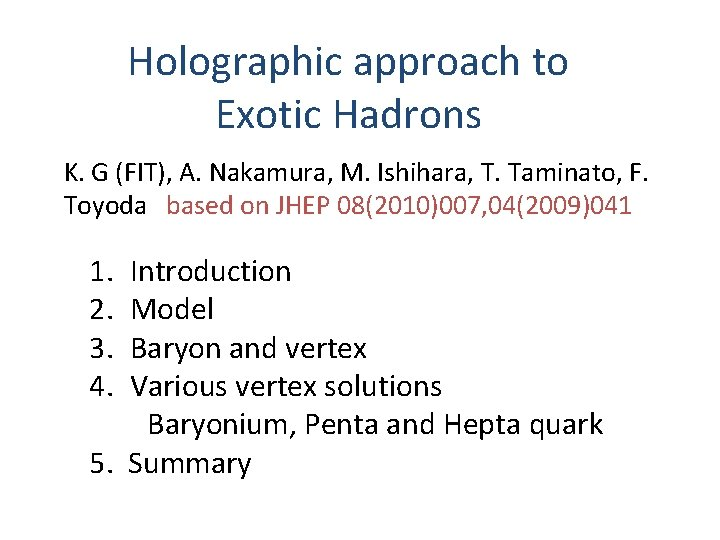Holographic approach to Exotic Hadrons K. G (FIT), A. Nakamura, M. Ishihara, T. Taminato, F. Toyoda　based on JHEP 08(2010)007, 04(2009)041 1. 2. 3. 4. Introduction Model Baryon and vertex Various vertex solutions Baryonium, Penta and Hepta quark 5. Summary1. Introduction 　based on Type IIB superstring • 　　Holographic “QCD” (YM Fields + Quarks) 　 a) SU(Nc) Yang-Mills Theory 　　　　　 10 D gravity with D 3 branes 　　　　 　　　　　　　 Closed strings Open Strings (=Large)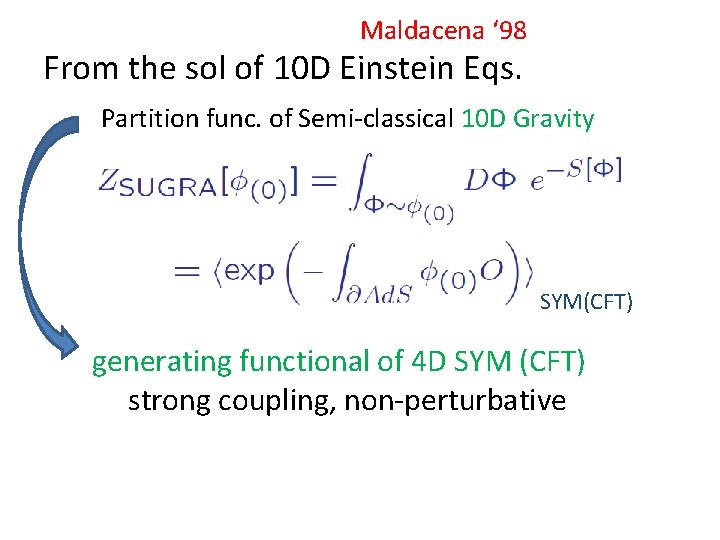Maldacena ‘ 98 From the sol of 10 D Einstein Eqs. Partition func. of Semi-classical 10 D Gravity SYM(CFT) generating functional of 4 D SYM (CFT) strong coupling, non-perturbative• b) Quarks　（by flavor branes, probe = Quenched Approxi. ) Karch-Katz JHEP 2003 Nf<<Nc flavor Color ・Color superconductor n. B large Nc ≈ Nf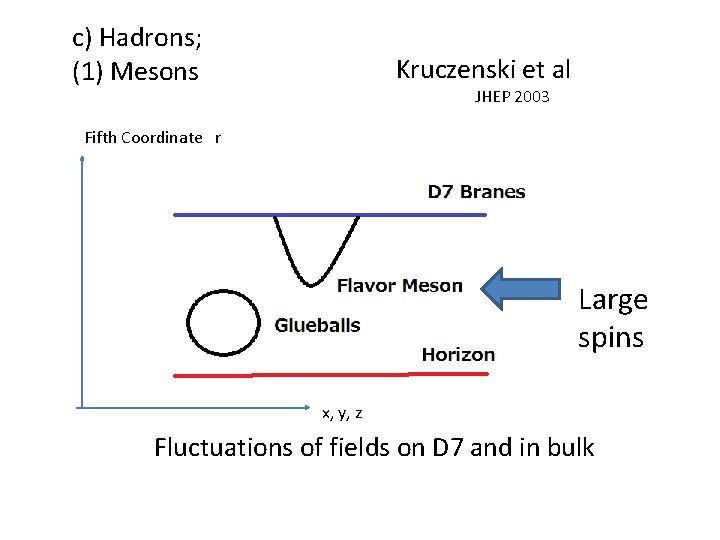c) Hadrons; (1) Mesons Kruczenski et al JHEP 2003 Fifth Coordinate r Large spins x, y, z Fluctuations of fields on D 7 and in bulk(2)Baryons: Vertex (D 5 Brane)+ Nc Strings Witten 1998 Nc-string as U(1) flux Exotic Hadorons appear through the structure of D 5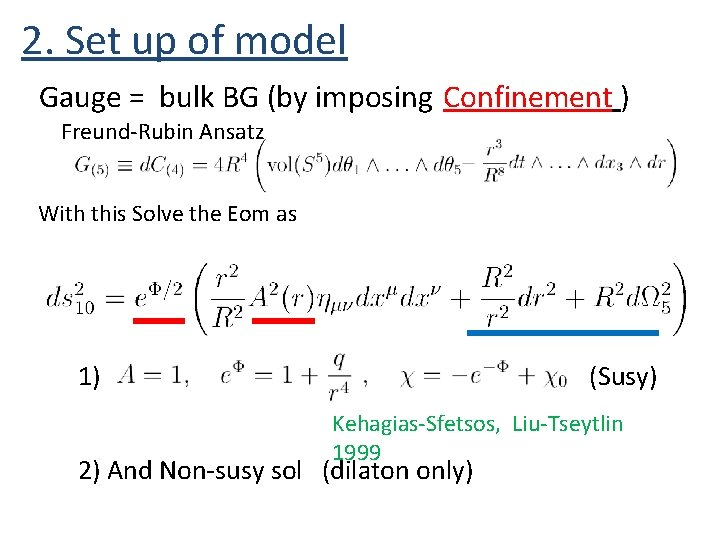2. Set up of model Gauge = bulk BG (by imposing Confinement ) Freund-Rubin Ansatz With this Solve the Eom as 1) (Susy) Kehagias-Sfetsos, Liu-Tseytlin 1999 2) And Non-susy sol　(dilaton only)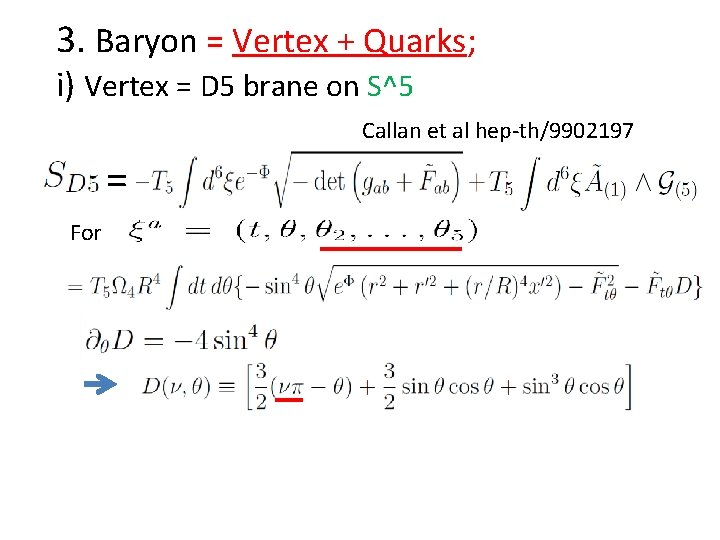3. Baryon = Vertex + Quarks; i) Vertex = D 5 brane on S^5 Callan et al hep-th/9902197 = ForEnergy density U After Legendre tr. W. r. t. to D Solution has generally two cusps at (north and south poles)Meaning of ν : separation of outgoing flux (1 -ν)N strings νN strings : for a unit string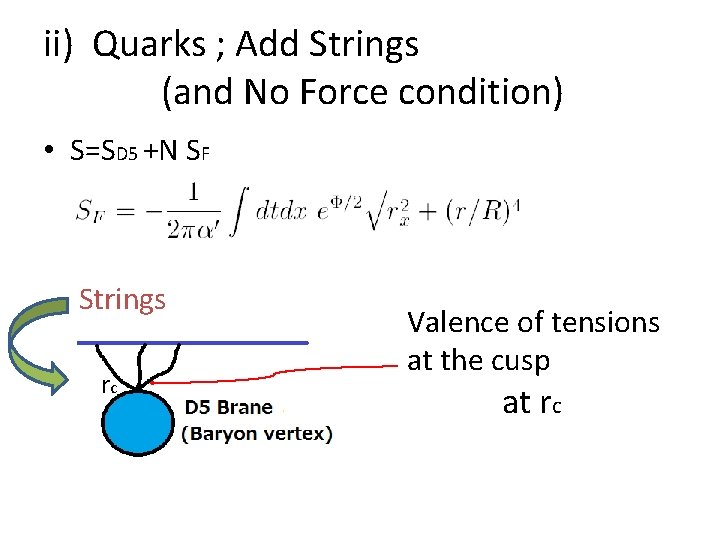ii) Quarks ; Add Strings (and No Force condition) • S=SD 5 +N SF Strings rc Valence of tensions at the cusp at rcNo force condition at rc for a point (x’=0) baryon config. For D 5 : For SF :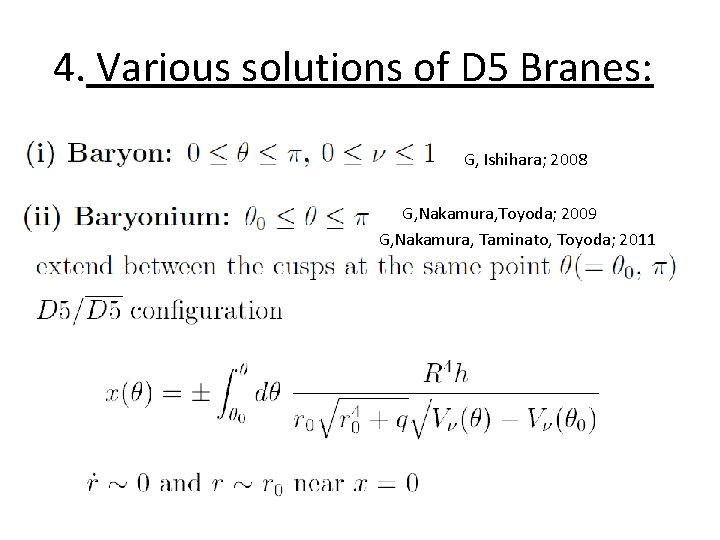4. Various solutions of D 5 Branes: G, Ishihara; 2008 G, Nakamura, Toyoda; 2009 G, Nakamura, Taminato, Toyoda; 2011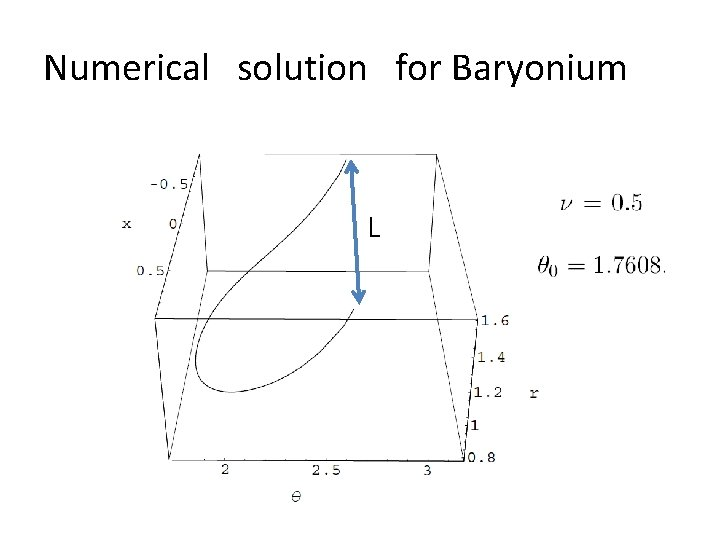Numerical　solution　for Baryonium L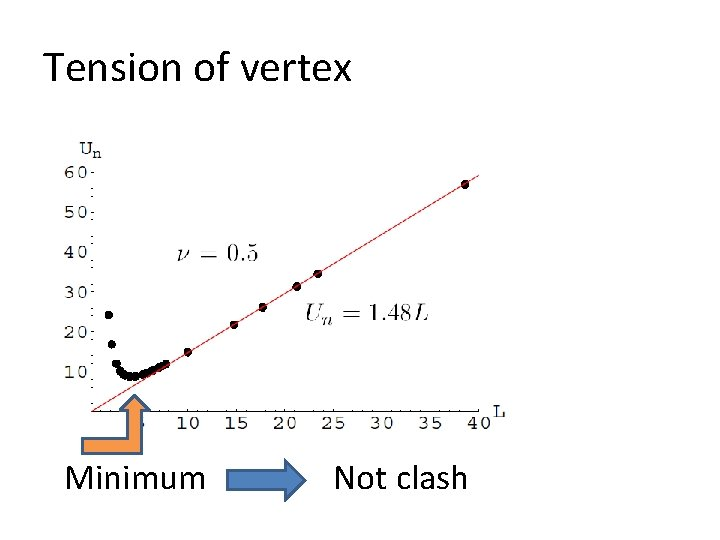Tension of vertex Minimum Not clash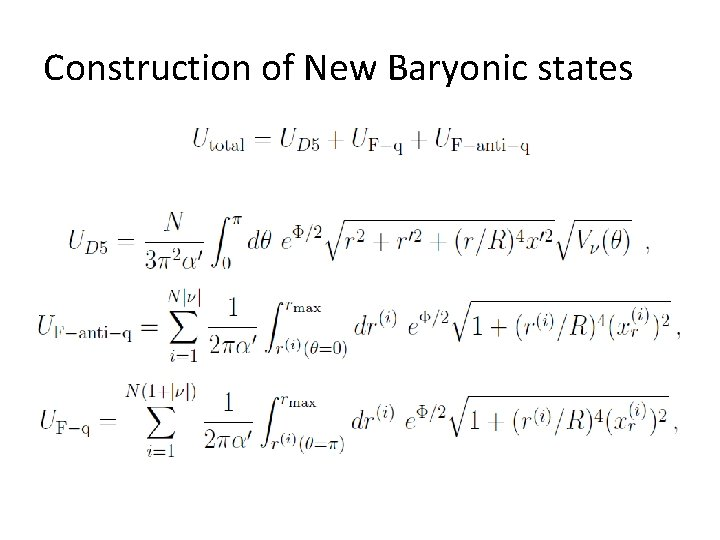Construction of New Baryonic states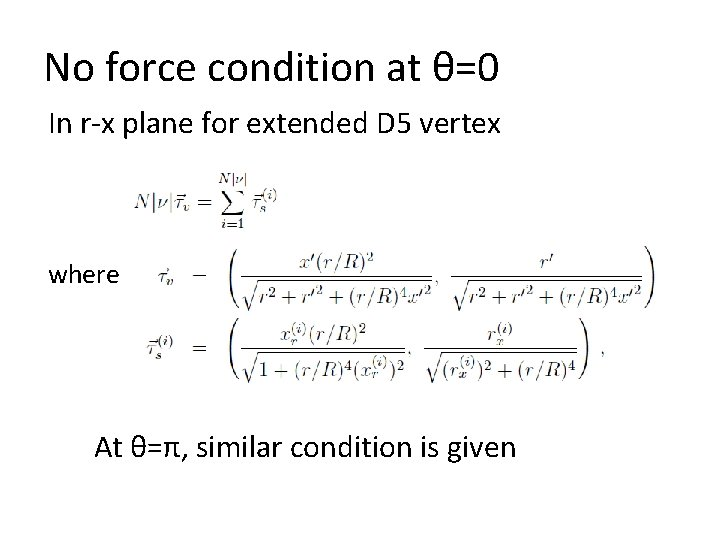No force condition at θ=0 In r-x plane for extended D 5 vertex where At θ=π, similar condition is given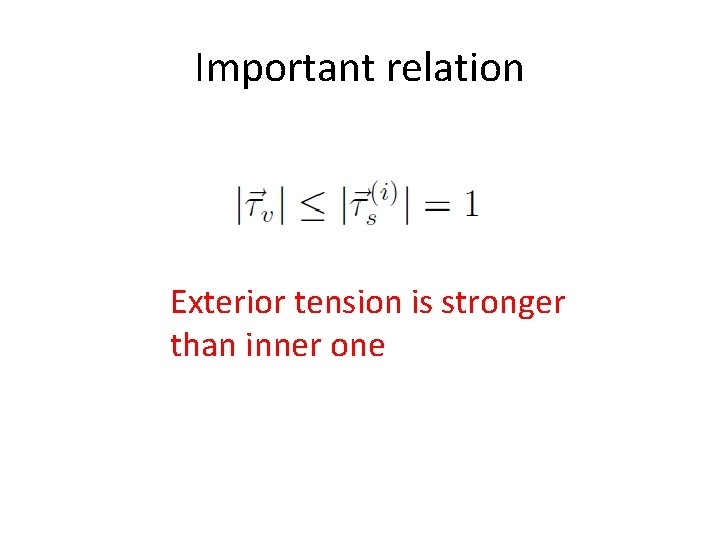Important relation Exterior tension is stronger than inner onePenta Quark (for N=3) Consider a configuration of At θ=0 θ=π r=0 Infinite energy Difficult to realize this type (Penta-Quark)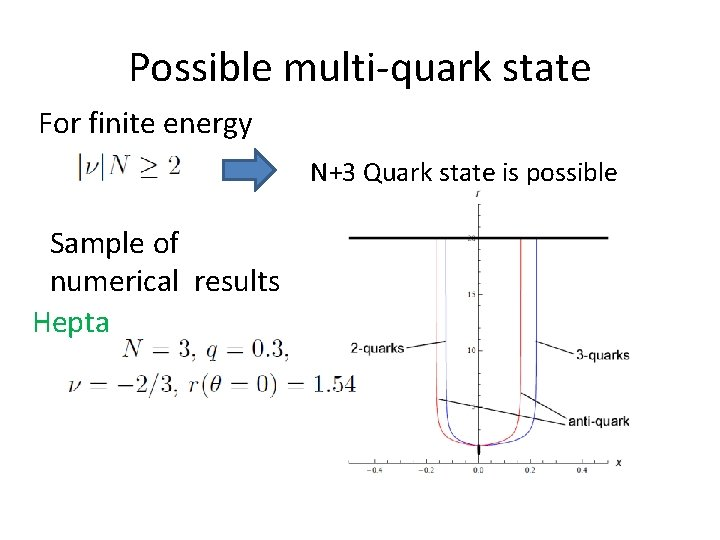Possible multi-quark state For finite energy N+3 Quark state is possible Sample of numerical results HeptaCounting rule for baryonic states Mass of nq quark state Lowest energy of the multiquark statesState with extended vertex : split type Energy is given by two kinds of tensions; 1. String 2. Vertex energy is approximated as τD 5=　 For Hepta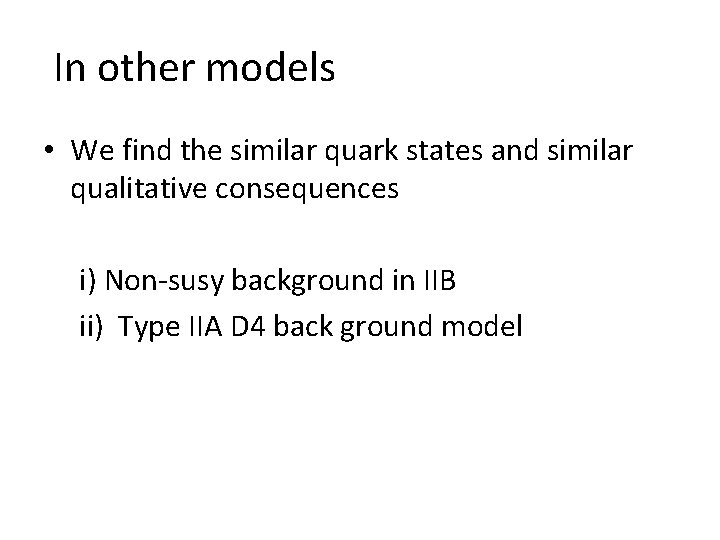In other models • We find the similar quark states and similar qualitative consequences i) Non-susy background in IIB ii) Type IIA D 4 back ground model5. Summary • • • Baryonic penta quark may be excluded Hepta quark is possible Baryonium –tetra quark state is stable Their mass obey the quark counting rule +vertex tension would be seen for extended vertex state (evidence of String theory)